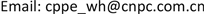﻿ 哈萨克斯坦与中国天然气管道管输费定价机制异同分析 Analysis of Pricing Mechanism of Natural Gas Pipeline Transportation Tariff between Kazakhstan and China

Journal of Oil and Gas Technology
Vol. 41  No. 04 ( 2019 ), Article ID: 32078 , 4 pages
10.12677/JOGT.2019.414065

Analysis of Pricing Mechanism of Natural Gas Pipeline Transportation Tariff between Kazakhstan and China

Hong Wang1, Yi Liu1, Anqi Wang2, Yating Zhu2

1China Petroleum Pipeline Engineering Co. Ltd., Langfang Hebei

2China Petroleum Pipeline Engineering Co. Ltd. International, Langfang HebeiReceived: Feb. 9th, 2019; accepted: Apr. 15th, 2019; published: Aug. 15th, 2019ABSTRACT

There were many differences in the pricing mechanism of natural gas pipelines between Kazakhstan and China in terms of laws, regulations and calculation methods. This paper introduces the development of natural gas pipeline network in Kazakhstan and its pricing mechanism for pipeline transportation tariff, analyzes and compares the differences in calculation methods and results between Kazakhstan and China.

Keywords:Natural Gas Pipeline, Transportation Price, Pricing Tariff Mechanism

1中国石油管道局工程有限公司，河北 廊坊

2中国石油管道局工程有限公司国际事业部，河北 廊坊1. 引言

2. 哈萨克斯坦天然气管网发展

3. 哈萨克斯坦天然气管输费定价机制

$管输单价=\frac{营业收入}{年周转量}$ (1)

$营业收入=总成本费用+\frac{准许收益}{1-企业所得税率}$ (2)

$准许收益=监管资产基础×准许收益率$ (3)

$利润率=债务成本×\left(1-企业所得税率\right)×\frac{债务资本}{资本总额}+股权成本×\frac{股权资本}{资本总额}$ (4)

$债务成本=\left(1+银行间同业拆借利率+利差\right)×\frac{1+哈国通胀率}{1+美国通胀率}-1$ (5)

$股权成本=\left(1+行业收益率+国家风险溢价\right)×\frac{1+哈国通胀率}{1+美国通胀率}-1$ (6)

4. 中国天然气管输费定价机制

$管道运价率=\frac{年度准许总收入}{年度总周转量}$ (8)

$年度准许总收入=准许成本+准许收益+税费$ (9)

$准许成本=运行维护费+折旧+摊销$ (10)

$准许收益=有效资产×准许收益率$ (11)

$有效资产=固定资产净值+无形资产净值+营运资本$ (12)

$营运资本=运行维护费×20%$ (13)

$所得税=准许收益×\left(1-资产负债率\right)×\frac{所得税率}{1-所得税率}$ (14)

5. 中、哈管输费定价机制异同分析

6. 结语

 黄伟, 杨桂荣, 张品先. 哈萨克斯坦石油天然气工业发展现状及展望[J]. 天然气与石油, 2015, 33(2): 1-8.

 石凯, 黄永章. 浅析中哈原油管道管输费的定价策略[J]. 国际石油经济, 2011, 19(10): 51-54.

 赵连增, 杜敏, 芮旭涛. 中国天然气管道运输价格管理新机制解读——《天然气管道运输价格管理办法(试行)》剖析之一[J]. 国际石油经济, 2017, 25(2): 16-22.

[编辑] 孙巍